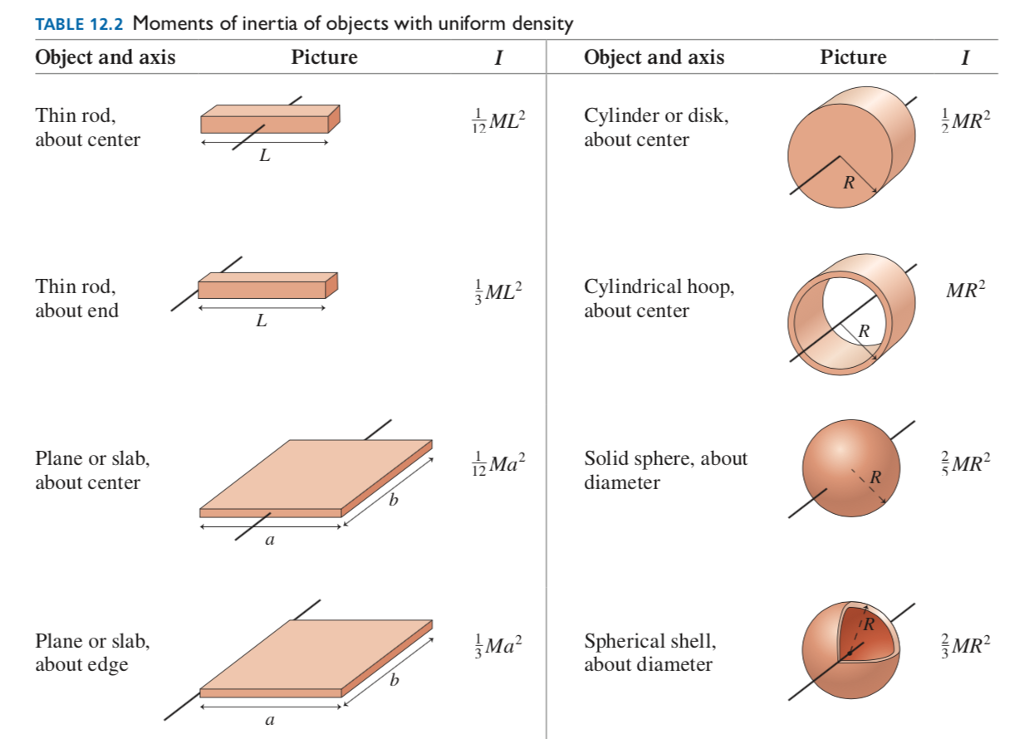# Problem: Derive the following:

###### FREE Expert Solution

The moment of inertia of an object can be defined as:

$\overline{){\mathbf{I}}{\mathbf{=}}\mathbf{\sum }_{\mathbf{i}}{{\mathbf{m}}}_{{\mathbf{i}}}{{{\mathbf{r}}}_{{\mathbf{i}}}}^{{\mathbf{2}}}}$

85% (67 ratings)###### Problem Details

Derive the following:Frequently Asked Questions

What scientific concept do you need to know in order to solve this problem?

Our tutors have indicated that to solve this problem you will need to apply the Moment of Inertia via Integration concept. You can view video lessons to learn Moment of Inertia via Integration. Or if you need more Moment of Inertia via Integration practice, you can also practice Moment of Inertia via Integration practice problems.